# Sample Question Paper - 8 Notes | Study Sample Papers For Class 9 - Class 9

## Class 9: Sample Question Paper - 8 Notes | Study Sample Papers For Class 9 - Class 9

The document Sample Question Paper - 8 Notes | Study Sample Papers For Class 9 - Class 9 is a part of the Class 9 Course Sample Papers For Class 9.
All you need of Class 9 at this link: Class 9

Section - A

Q.1. A player caught a cricket ball of mass 0.15 kg moving at a rate of 20 ms-1. If the catching process be completed in 0.1 s, the force of the blow exerted by the ball on the hands of the player is
(a) 0.3 N
(b) 30 N
(c) 3 N
(d) 300 N
Ans.
(c) Here m = 0.15 kg, u = 20 m s-1, v = 0 and t = 0.1 s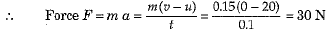Q.2. How would you confirm that a colourless liquid given to you is pure water ?
Ans.
By evaporating the colourless liquid on a low flame. If there is no residue left, then the colourless liquid is pure water.

Q.3. Which one of the following is not important for individual health?
(a) Living in clean space.
(b) Good economic condition.
(c) Social equality and harmony.
(d) Living in a large and well furnished house.
OR
We should not allow mosquitoes to breed in our surroundings because they
(a) multiply very fast and cause pollution.
(b) are vectors for many diseases.
(c) bite and cause skin diseases.
(d) are not important insects.
Ans.
(d) Living in a large and well furnished house.
Explanation: The conditions essential for good health are clean surrounding, availability of clean drinking water, availability of clean adequate, nutritious food and social equality and harmony.
OR
(b) Are vectors for many diseases.
Explanation: Many animals which live with us carry disease. These animals transfers infectious agents from sick person to another potential host (healthy person) and act as vectors. Mosquitoes are known to act as vector for many diseases. For example, Mosquito Anopheles, Culex and Aedes causes malaria, filariasis and dengue respectively.

Q.4. The SI unit of frequency is
(a) second (s)
(b) hertz (Hz)
(c) decibel (dB)
(d) watt/(metre)2 (W m-2)
Ans.
(b) SI unit of frequency is 1 hertz (1 Hz), where 1 Hz = 1 cycle per second.

Q.5. If the displacement of an object is proportional to square of time; then the object moves with :
(a) uniform velocity
(b) uniform acceleration
(c) increasing acceleration
(d) decreasing acceleration
Or
The speed-time graph of a body is a straight line parallel to time axis. The body has:

(a) uniform acceleration
(b) uniform speed
(c) variable speed
(d) variable acceleration
Ans.
(b) Or (b)

Q.6. Find out the wrong statement from the following:
(a) White revolution is meant for increase in milk production.
(b) Blue revolution is meant for increase in fish production.
(c) Increasing food production without compromising with environmental quality is called as sustainable agriculture
(d) None of the above
Ans.
(d)
Explanation: All the statements are correct. White revolution is meant for increase in milk production, blue revolution is meant for increase in fish production and green revolution is for high production of food grains. Sustainable agriculture is the increasing food production without compromising with environmental quality.

Q.7. The number of atoms of hydrogen present in 17 g NH3 is
(a) 6.022 x 1023
(b) 6.022 x 1022
(c) 1.8066 x 1024
(d) 6.022 x 1024
Ans.
(c)

Q.8. Poultry fowl are susceptible to the following pathogens :
(a) Viruses
(b) Bacteria
(c) Fungi
(d) All of the above
Or
When we breathe in air, nitrogen also goes inside along with oxygen. What is the fate of this nitrogen?
(a) It moves along with oxygen into the cells
(b) It comes out with the CO2 during exhalation
(c) It is absorbed only by the nasal cells
(d) Nitrogen concentration is already more in the cells so it is not at all absorbed
Ans.
(d) or (b)

Q.9. Which of the following would weigh the highest?
(a) 0.2 mole of sucrose (C12H22O11)
(b) 2 moles of CO2
(c) 2 moles of CaCO3
(d) 10 moles of H2O
Ans.
(c) 2 moles of CaCO
Explanation: 2 moles of CaCO3 would weigh the highest. It is because,
(a) Mass of 1 mole of sucrose (C12H22O6) = (12 x 12) + (1 x 22) + (16 X 11)= 342 g
Mass of 0.2 mole of sucrose = 342 x 0.2 = 68.4 g
(b) Mass of 1 mole of CO2 = 12 + (16 x 2) = 44 g
Therefore, mass of 2 moles of CO2 = 44 x 2 = 88 g
(c) Mass of 1 mole of CaCO3 = 40 + 12 + (16 x 3) = 100g
Mass of 2 moles of CaC03 = 100 x 2 = 200 g
(d) Mass of 1 mole of H2O = 2 + 16 = 18 g
Mass of 10 moles of H2O = 18 X 10 = 180 g
Therefore, mass of 2 moles of CaCO3 is the highest, i.e., 200 g.

Q.10. What would happen, if all the oxygen present in the environment is converted to ozone ?
(а) We will be protected more.
(b) It will become poisonous and kill living forms.
(c) Ozone is not stable, hence it will be toxic.
(d) It will help harmful sun radiations to reach earth and damage many life forms.
Ans.
(b) If all the oxygen present in the environment is converted into ozone, it will be difficult to breathe and survive. All the living forms would be killed.

Q.11. Which one of the following is a correct electronic configuration of sodium ?
(a) 2,8
(b) 8,2,1
(c) 2,1,8
(d) 2,8,1
Ans.
(d)

Q.12. How can we separate a mixture of two immiscible liquids
Ans.
By using a separating funnel.

Q.13. State whether the following statements are True or False :
Inertia of an object depends on its mass as well as shape and size.
Ans.
False - Inertia of an object depends on its mass only.

Q.14. Assertion : A sound created in a big hall will persist by repeated reflection from the walls until it is reduced to a value where it is no longer audible.
Reason : Sound undergoes reflection.
(i) A
(ii) B
(iii) C
(iv) D
Ans.
(i)

Section - B

Q.15. The velocity-time graph of an object is shown in the figure given below.
(a) State the kind of motion that object has from A to B and from B to C.
(b) Identify the part of graph where the object has zero acceleration. Give reason for your answer.
(c) Identify the part of graph where the object has negative acceleration.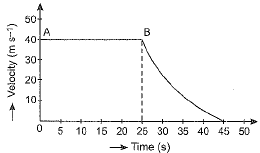Or
A motorcyclist riding motorcycle A, who is travelling at 36 km h-1, applies the brakes and stops the motorcycle in 10 s. Another motorcyclist o f motorcycle B, who is travelling at 18 km h-1, applies the brakes and stops the motorcycle in 20 s. Plot speed-time graphs for the two motorcycles. Which of the two motorcycles travelled farther before it came to a stop ?
Ans.
(a) Region AB of graph shows uniform motion but the region BC shows non-uniform motion.
(b) In region AB of the graph the object has zero acceleration because velocity in this region is constant at 40 ms-1.
(c) In region BC the object has negative acceleration because the velocity is falling from 40 ms-1 at point B (time = 25 s) to zero at point C (time = 45 s).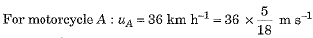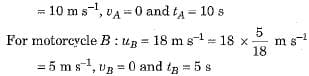Speed-time graphs for motorcycles A and B have been plotted in figure.
Distance covered by motorcycle A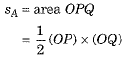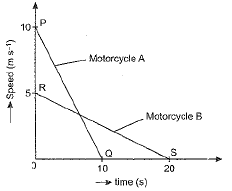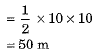and distance covered by motorcycle B
sB = area ORS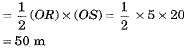Thus, both motorcycles travelled equal distance before coming to a stop.

Q.16. In a reaction, 5.3 g of sodium carbonate reacted with 6 g of ethunuic acid. The products were 2.2 g of carbon dioxide, 0.9 g water and 8.2 g of sodium ethanoate. Show that these observations are in agreement with the law of conservation of mass, sodium carbonate + ethanoic acid → sodium ethanoate + carbon dioxide + water.
Or
Cinnabar (HgS) is a prominent ore of mercury. How many grams of mercury is present in 225 g of pure HgS ? Molar mass of Hg and S are 200.6 g mol-1 and 32 g mol-1 respectively.
Ans.
(a) Mass of reactants before experiment
= Mass of sodium carbonate + Mass of ethanoic acid
= 5.3 g + 6 g = 11.3 g
Mass of products after experiment
= Mass of sodium ethanoate + Mass of carbon dioxide + Mass of water
= 8.2 g + 2.2 g + 0.9 g = 11.3 g
The mass of reactants is equal to the mass of products, therefore, it proves law of conservation of mass.
Or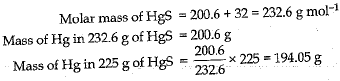Q.17. What type of motion is represented by each of the following graphs ?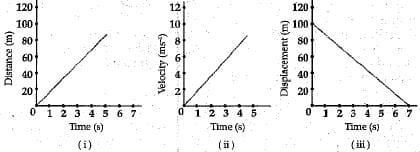OR
Which law of motion can best describe the following:
(i) Force applied while pulling a lawn mower.
(ii) Shoulder bone fracture from recoil of a gun.
(iii) Coin remains on the table, when table cloth is suddenly removed.

Ans. (i) Uniform motion.
(ii) Uniform accelerated motion,
(iii) Uniform motion.
OR
(i) According to Newton's II law of motion
(ii) According to Newton's III law of motion
(iii) According to Newton's I law of motion.

Q.18. State two factors that determine the rate of diffusion of a liquid into another liquid.
Or
A substance A has high compressibility and can be easily liquefied. It can take the shape of any container. Predict the nature of the substance. Enlist four properties of this state of matter.

Ans. The two factors are :
Miscibility : Greater the miscibility of one liquid into the other, greater will be rate of diffusion.
Temperature : With increase in temperature, the kinetic energy of particles increases, hence the rate of diffusion increases.
Or
The substance A is a gas because gases have large empty spaces between the particles. It has high compressibility and can be easily liquefied.
Properties of gases :
(i) Gases have no fixed shape and volume.
(ii) Gases can be compressed on applying pressure to give liquids.
(iii) Particles of a gas are moving in all possible directions with all possible speeds.
(iv) Gases show the property of diffusion.

Q.19. In brief state what happens when :
(a) dry apricots are left for sometime in pure water and later transferred to sugar solution?
(b) a red blood cell is kept in concentrated saline solution ?
(c) the plasma membrane of a cell breaks dowm ?
(d) rheo leaves are boiled in water first and then a drop of sugar syrup is put on it ?
(e) golgi apparatus is removed from the cell ?
Ans.
(a) First it swells due to endosmosis and then exosmosis occurs and it shrinks.
(b) It will lose water and shrink.
(c) The cell will die.
(d) The cell gets killed on boiling, so no plasmolysis.
(e) All sorts of vesicle formation stops.

Q.20. (a) From the symbol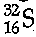state :
(i) Atomic number of sulphur
(ii) Mass number of sulphur
(iii) Electronic configuration of sulphur
(b) Which of the two elements given below would be chemically more reactive, 'X' of atomic number 18 or element 'Z' of atomic number 16 and why ?
Ans.
(a)
(i) 16
(ii) 32
(iii) 16S = 2,8,6
(b) Z is more reactive than X.
Electronic configuration of Z = 2, 8, b which may gain 2 electrons and thus is more reactive whereas X = 2, 8,8 has complete octet and is inert.

Q.21. (a) State the basic difference between atoms and molecules.
(b) Why does not atomic mass of an element represent the actual mass of its atoms ?
(c) “The atomic mass of an element is in fraction,” What does this mean ?
Ans. (c) Atom is the building block of all matter.
A molecule is in general a group of two or more atoms of the same or different elements that are chemically bonded.
(b) The actual mass of an atom is extremely small. We represent the mass of an atom as a relative mass compared to 1/12 of the mass of one carbon-12 atom.
(c) Many of the elements have isotopes with different masses. The atomic mass of an element is the average of the masses of these isotopes which comes out to be a fraction.

Q.22. (a) State the universal law of gravitation.
(b) Write the formula to find the magnitude of the gravitational force between the earth and an object on the surface of the earth.
Ans.
(a) Every particle in this universe attracts every other particle with a force which is directly proportional to the product of their and inversely proportional to the square of the distance between their centres.
(b) The formula is F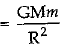, where M is the mass of the earth, m is the mass of the object on the surface of the earth and R is the radius of the earth.

Q.23. Ozone is poisonous and is found in upper atmosphere of earth yet the world is worried about its depletion. Explain why? Write any two methods to control ozone layer depletion.
Ans.
Ozone forms a protective layer and prevents harmful rays from entering the earth's surfaces and thereby harming life.
UV rays may cause skin cancer.
Methods to control ozone layer depletion are:
(i) Do not use CFC's.
(ii) Reduce the release of nitrogen oxides and sulphur oxides in nature.

Q.24. The given figure shows two crop fields (Plots A and B) which have been treated by manures and chemical fertilisers. Keeping other environmental factors same, observe the graph and answer the following questions :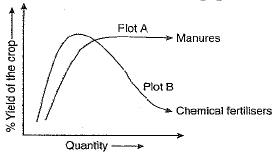(a) Why does plot B show sudden increase and then gradual decrease in the field ?
(b) Why is the height peak in A graph slightly delayed ?
(c) What is the reason for the different patterns of the two graphs ?

Or
(а) What are pesticides ? Give examples.
(b) Why one should avoid use of chemical pesticides ?
Ans.
(a) Plot B shows sudden increase and then gradual decrease as use of chemical fertilisers provide essential minerals immediately, resulting in good growth but in the long run, destroys soil fertility as organic matter in soil is not replenished and hence decreased yield.
(b) Delayed peak in Plot A as the organic manure releases minerals slowly.
(c) Organic manure improves soil fertility, soil composition, increasing its water holding capacity and hence continues to give good yield whereas chemical fertilisers do not help in improving soil quality and hence the nutrients got washed away with irrigation, resulting in decrease in yield after a peak.
Or
(a) The chemicals which are used to kill insect pests, fungi, weeds and rodents are called pesticides. Example : DDT, Malathion and BHC.
(b) Use of chemical pesticides should be avoided because they cause environmental pollution as these chemicals are non-biodegradable.

Section - C

Q.25. Two objects A and B, having masses 100 kg and 75 kg, moving with velocities 40 km/h and 60 km/h respectively. Answer the following :
(а) Which will have greater inertia ?
(b) Which will have greater momentum ?
(c) Which will stop first if equal negative acceleration is applied on both ?
(d) Which will travel greater distance in 10 minutes ?
(e) Which will impart greater force if collided with a wall ?
Ans.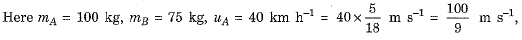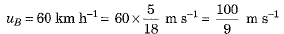(a) The object A has greater inertia because its mass is more.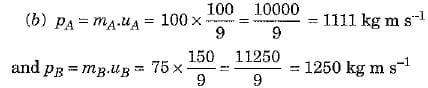Thus, object B has greater momentum.
(c) If equal negative acceleration - a is applied on both then object A will stop first because its initial velocity is less. As v - 0, hence we have 0 = u - at or t =u/a.
(d) As distance travelled in time t is given by s = ut + 1/2 at2. Since acceleration ‘a' is negative, the relation becomes s= ut - 1/2at2. Of course uB > uA but depending upon the value of 'a', sA may be less than or greater than sB.
(e) The force imparted on collision with a ball is equal to rate of change of momentum of the given object. As a result, object B will impart a greater force.

Q.26. (a) How are sol, solution and suspension different from each other
(b) (i) Conversion of solid to vapour is called sublimation, Name the term used to denote the conversion o f vapour to solid.
(ii) Conversion of solid state to liquid state is called fusion. What is meant by latent heat of fusion .
Ans.
(a)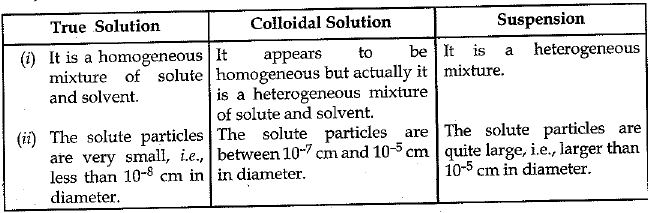(b) (i) Sublimation.
(ii) The amount of heat required to convert 1 kg of solid into liquid at one atmosphere pressure at its melting point is known as its latent heat of fusion.

Q.27. (a) Briefly explain Bohr-Bury scheme for the distribution of electrons in different shells.
(b) An atom has 2 electrons in its outermost shell M. What is the atomic number of the element ? Also mention its name
Ans.
(a) The following rules are followed for writing the number of electrons in different energy levels or shells :
(i) The maximum number of electrons present in a shell is given by the formula 2n2 where 'n' is the orbit number or energy level index 1,2,3....
Hence, the maximum number of electrons in different shells are as follows :
1st orbit or K-shell will be = 2 x 12 = 2,
2nd orbit or L-shell will be = 2 x 22 = 8,
3rd orbit or M-shell will be = 2 x 32 = 18,
4th orbit or N-shell will be = 2 x 42 = 32, and so on.
(ii) The maximum number of electrons that can be accommodated in the outermost orbit is 8.
(iii) Electrons are not accommodated in a given shell, unless the inner shells are filled. That is, the shells are filled in a step-wise manner.
(b) Atomic number of element = 12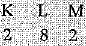Element is magnesium.

Q.28. Enlist one important function of each of the following :
(i) Plastids
(ii) Mitochondria
(iii) Cell wall
(iv) Plasma membrane
(v) RER.

Ans. One function of each :
(i) Plastids : They are specialised to synthesise and store organic substances.
(ii) Mitochondria : It is known as powerhouse of the cell. Cellular respiration takes place in this organelle resulting in release of energy.
(iii) Cell wall : It provides rigidity, permeability and protection to the plant cell.
(iv) Plasma membrane : It regulates entry and exit of molecules from within the cell to exterior and vice-versa.
(v) RER : It is the site of protein synthesis.

Q.29. (a) Define watt, Express kilowatt in terms of joule per second. A 150 kg car engine develops 500 W for each kg. What force does it exert in moving the car at a speed of 20 ms-1
(b) ) Compare the power at which each of the following is moving upwards against the force of gravity ?
(i) A butterfly of mass 1.0 g that flies upward at a rate of 0.5 ms-1.
(ii) A 250 g squirrel climbing up on a tree at a rate of 0.5 ms-1 .
Ans.
(a) The power of an agent is said to be one watt if it does one joule of work in one second.
1 kW = 1000 W = 103 J s-1
Given: m =150 kg, P = 500 W, v = 20 ms-1
Total power =150 x 500 = 75000 W
Hence,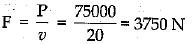(b) (i) P = Fv = mgv
= 1 x 10-3 x 10 x 0.5
= 5 x 10-3 W
(ii) P = Fv = mgv
= 250 x 10-3 x 10 x 0.5.
= 1.25 W

Q.30. (i) Define density.
(ii) Density of a substance is one of its characteristic properties. Explain.
(iii) State unit of density in SI.
(iv) How can we identify whether the substance is pure or not by knowing its density ?
Ans.
(i) Mass per unit volume is density.
(ii) The density of a given substance, arises from the internal arrangement of its atoms which make to determine it a characteristic property. It is different for different substances.
(iii) One can identify the purity of substance by knowing the density of the substance. kg/m3.
(iv) Density of pure substance is constant. Thus by comparing the observed density and exact density, we can determine whether the substance is pure or not.

The document Sample Question Paper - 8 Notes | Study Sample Papers For Class 9 - Class 9 is a part of the Class 9 Course Sample Papers For Class 9.
All you need of Class 9 at this link: Class 9Use Code STAYHOME200 and get INR 200 additional OFF

### Up next## Sample Papers For Class 9

191 docs|12 tests

### Up nextTrack your progress, build streaks, highlight & save important lessons and more!

,

,

,

,

,

,

,

,

,

,

,

,

,

,

,

,

,

,

,

,

,

;